surveying indirect leveling

# surveying indirect leveling### Le nivellement topographie direct et indirect en Cas ou le point est inaccessible

Indirect leveling
1. Case where the point is accessible

1.1 Principe

Trigonometric indirect topography meveming make it possible to determine the height difference ΔH entre la station Td’un théodolite et un point P. This is done by measuring the inclined distance alog the line of sight Di and zenith angle denoted V in the figure opposite. From the diagram, we can write that: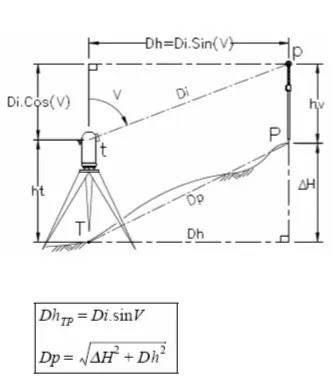ΔHTP is the height difference from Tto P
ht is the station height (or trunnion height).
hv is the height of the indicator or more generally the height targeted above the point sought (you can also put a surveying staff at P).
We deduce the horizontal distance Dh
We deduce the distance according to the slope Dp :
There are 2 cases which depend on whether or not it is possible to measure the distance Dp.1.2 Case where the distance along the slope Dp is measurable

C’est le cas si le terrain présente une pente régulière entre S et A. On peut alors mesurer directement la distance Dp à la chaîne avec une précision correcte.
La méthode est la suivante : depuis le théodolite stationné en S, l’opérateur vise la mire topographie en interceptant la graduation correspondante la hauteur des tourillons ht de sorte que la visée soit parallèle à la droite SA dont l’opérateur a mesuré la longueur Dp. IL lit l’angle V correspondant, il mesure Dp et en déduit que :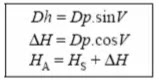1.3 Case where the distance following the slope Dp is not measurable

This is the case if the slope is uneven, on strongly bumpy ground, for exemple, if there are obstacles, etc.
it is necessary to calculate the hoirizontal distance Dh from the station S from the readings on a surveying rod placed at A.Dh is determined bt stadiometer from the m1, m2 and V readings.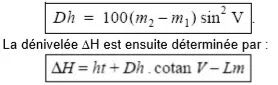2. Case where the point is inaccessibleWe seek to know the altitude of an inaccessible point C knowing only the altitude of close point A which will serve as a reference. If we can measure the horizontal distance AC, we can calculate the height difference from A to C by measuring the angle V read from A to C and the height of the axis of the trunnions ht. The altitude
is given by :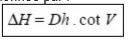A solution to get the horizontal distance Dh is to create a base AB by adding a second point B, to measure  the horizontal angles CBA and CAB.
This ^makesb it possible to solve the triangle ABC and therefore to calculaate the horizontal distances AC and BC. The altitude of C is then:We can also calculate the altitude of C twice: :
a firstg time from A
And a second from B and this control the result.
For this, it is necessary to determine the  ht à chaque station.
3. Applications

3. 1 Exercise n°1 :
Elevation of point  MThe distance along the slope (Am) can be measured.

Itis obtained by measuring the distance along the slop Am, m being on the ground plumb with M . The measurement gives : dAm = 43.42m

By means of a theodoliten stationed at A , you read the vertiucal angles of the sights on M and m. the altitude of station point A is : 125.63m.

Calculate the hjeight difference between A and m, deduce the horizontal distance Dh then calculate the height Mm as well as the altitude of point M

3.2 Exercise n°2 :
Elevation of point NWe cannot measure the distance along the slope (An), not being the plumb of N. A method allowing to remove this difficulty is to choose a base AB from which the point N is visible, to measure the horizontal distance AB as well as the angles NAB and NBA.
From these measurement we can solve t-he triangle ANB and deduce the horizontal distance AN that we cannot measure.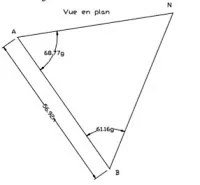You therfor make a second station at a point B chosen such that tghe triangle ANB is approximately equilateral.

3.3 Exercise n°3 :
Verification measures

In fact the distance 56.92m was measured along the slope AB .
For verification, you also note the following, values :

From the station at A, read the vertical angle at point B.
From the station in B, read the vertical angle on A and N.

Repear the calculations from A znf check them from B. You Will calculate the akltitude of B on the way .